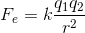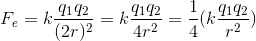# SAT II Physics : Coulomb's Law

## Example Questions

### Example Question #3 : Coulomb's Law

If the distance between two charged particles is doubled, the strength of the electric force between them will __________.

double

remain unchanged

be quartered

be halved

be quartered

Explanation:

Coulomb's law gives the relationship between the force of an electric field and the distance between two charges:The strength of the force will be inversely proportional to the square of the distance between the charges.

When the distance between the charges is doubled, the total force will be divided by four (quartered).